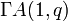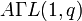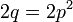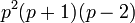# Element structure of general semiaffine group of degree one over a finite field

## Contents

This article gives specific information, namely, element structure, about a family of groups, namely: general semiaffine group of degree one.
View element structure of group families | View other specific information about general semiaffine group of degree one

This article describes the element structure of the general semiaffine group of degree one over a finite field of size$q$, where$q = p^r$, with$p$ the field characteristic and$r$ a positive integer. This group is denoted$\Gamma A(1,q)$ or$A\Gamma L(1,q)$.

Note that if$r = 1$, the group is the same as the general affine group of degree one$GA(1,q)$.

## Summary

Item Value
order$rq(q - 1)$

### Number of conjugacy classes formulas

For every fixed value of$r$, the number of conjugacy classes simply becomes a polynomial in$p$. The values of these polynomials for small$r$ are listed below:$r$ Polynomial in$p$ giving number of conjugacy classes
1$p$
2$p(p + 3)/2$

## Conjugacy class structure

### Case r = 2

We have$q = p^2$. In our discussion, we distinguish between conjugacy classes in the additive group, conjugacy classes in GA(1,q) that are outside the additive group, and conjugacy classes outside GA(1,q). Note that the additive group has size$q = p^2$, the group$GA(1,q)$ contains it and has size$q(q - 1) = p^2(p^2 - 1)$, and the whole group has size$2q(q - 1) = 2p^2(p^2 - 1)$.

Nature of conjugacy class Size of conjugacy class Number of such conjugacy classes Total number of elements
identity element of group, acts as identity map 1 1 1
non-identity element, in additive group. Acts as translation$q - 1 = p^2 - 1$ 1$q - 1 = p^2 - 1$
outside the additive group, but in GA(1,q) and the multiplicative part is in the prime subfield. Acts as$x \mapsto ax + b$, with$a \in \mathbb{F}_p^\ast \setminus \{ 1 \}$$q = p^2$$p - 2$$p^2(p - 2)$
outside the additive group, but in GA(1,q) and the multiplicative part is outside the prime subfield. Acts as$x \mapsto ax + b$, with$a \in \mathbb{F}_q^\ast \setminus \mathbb{F}_p^\ast$$2q = 2p^2$$p(p - 1)/2$$p^3(p - 1)$
outside GA(1,q), has order two (i.e., generates a permutable complement to the subgroup of index two that is GA(1,q))$p(p + 1)$ 1$p(p + 1)$
outside GA(1,q), its image mod the additive group has order two but it does not itself have order two$p (p - 1)(p + 1) = p^3 - p$ 1$p(p - 1)(p + 1) = p^3 - p$
outside GA(1,q), its image mod the additive group does not have order two$p^2(p + 1)$$p - 2$$p^2(p + 1)(p - 2)$
Total --$p(p + 3)/2 = (p^2 + 3p)/2$ (equals number of conjugacy classes in the group)$2p^2(p^2 - 1)$ (equals order of the whole group)